# 小中研究室

### Prediction of Rugby World Cup 2019 based on official World Rugby Rankings | konakalab

This article describes a prediction of Rugby World Cup 2019 (RWC2019), which will be held in the next September. This prediction model is using the official World Rugby Rankings. The ranking points used here is the 9/9 edition.
The derivation of the mathematical prediction model is explaind in the latter half of this article.

#### Tournament prediction

The figure below show the probabilities for the knodkout stage. The width of the strips shows the probabilities.

#### Pool stages

40 matches in the pool stages are predicted. The bar lengths denote the probabilities for each points. The matches are sored by the schaduled dates. The probabilities are for the teams witten in the left-hand side.
The probability distributons of the points for every team are also shown.
##### Pool A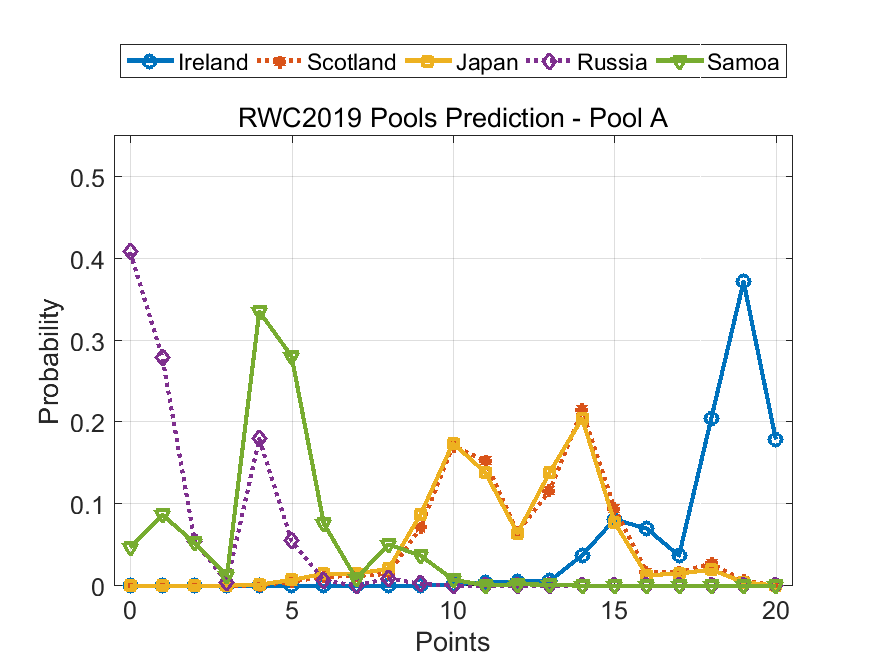##### Pool B
• note: NZ = New Zealand, SA = South Africa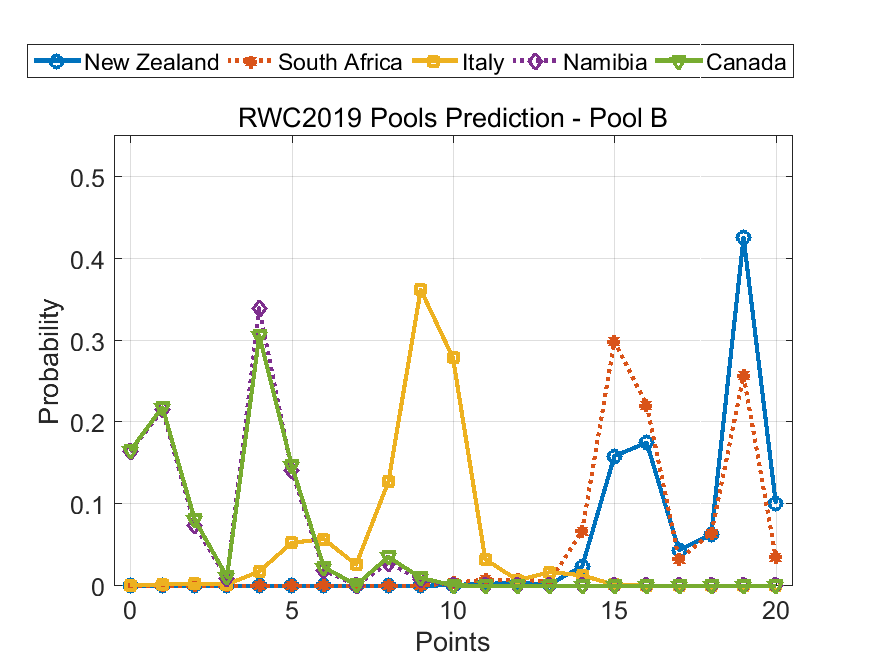##### Pool C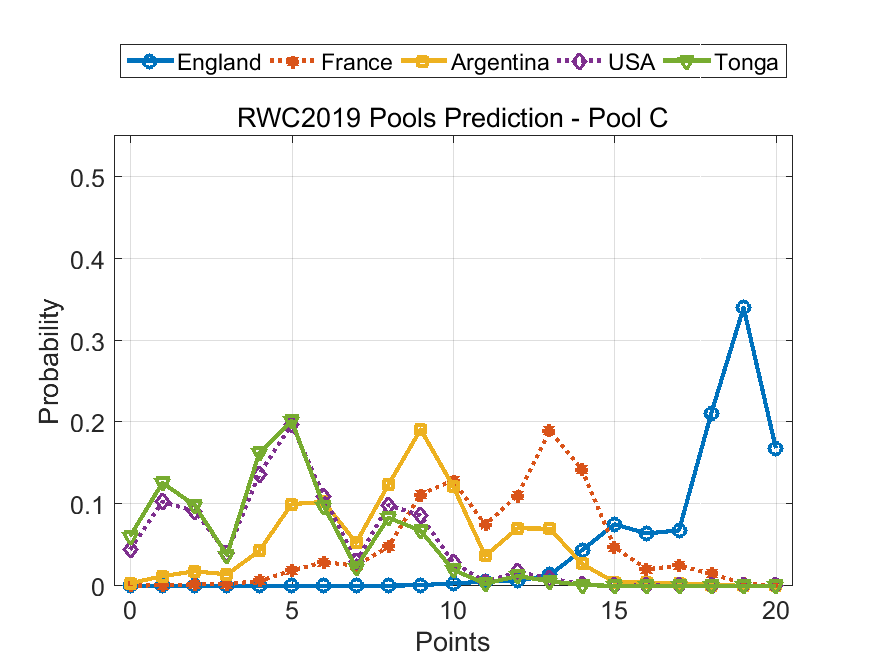##### Pool D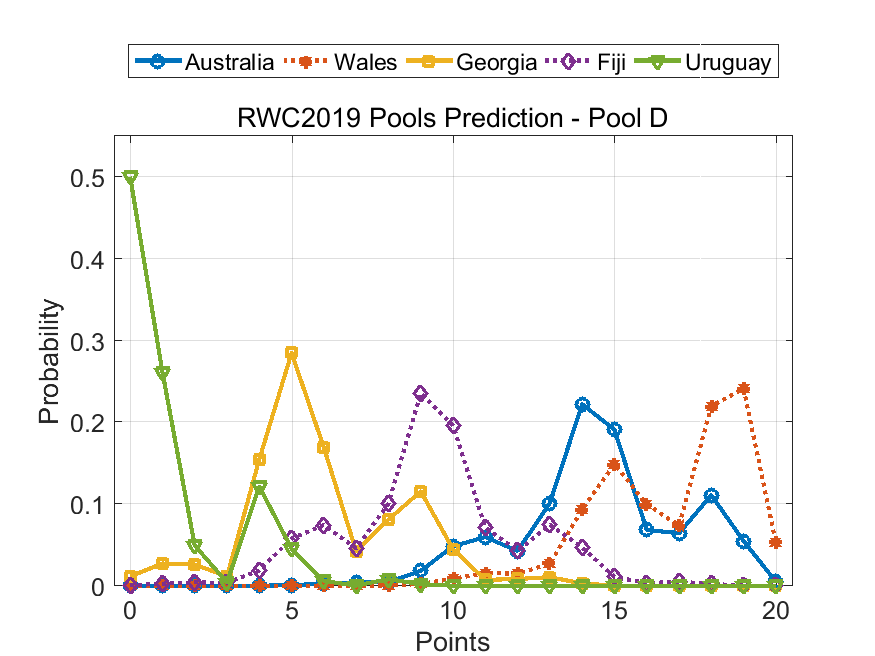#### Prediction model

The official World Rugby Rankings has strong correlaton with the match outcome. The figure below shows the relation between the ranking point difference and the score difference in the last four RWCs (2003 to 2015).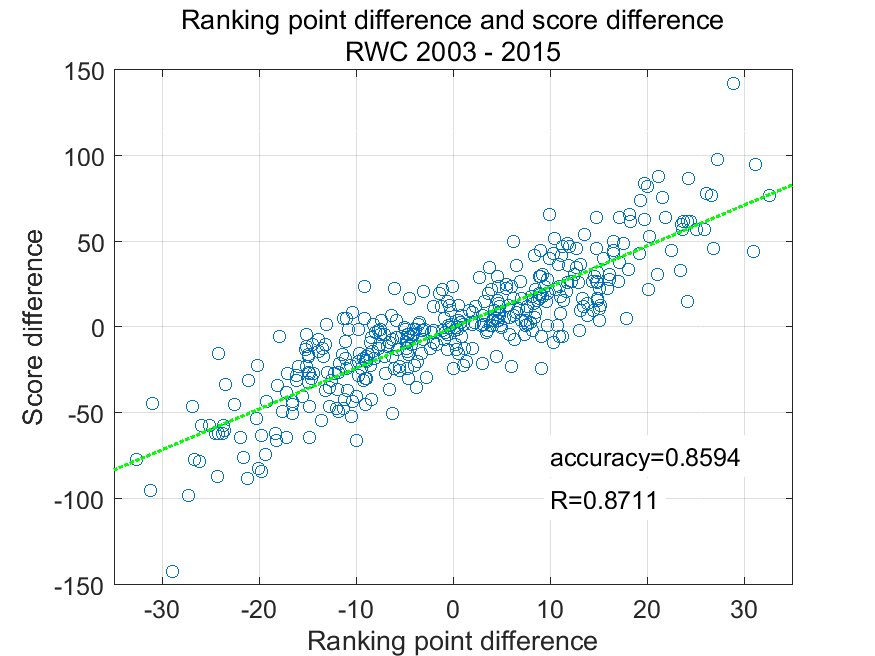By introducing an assumption on recovery from fatigue after full-match, the prediction accuracy from the ranking points could be maximized.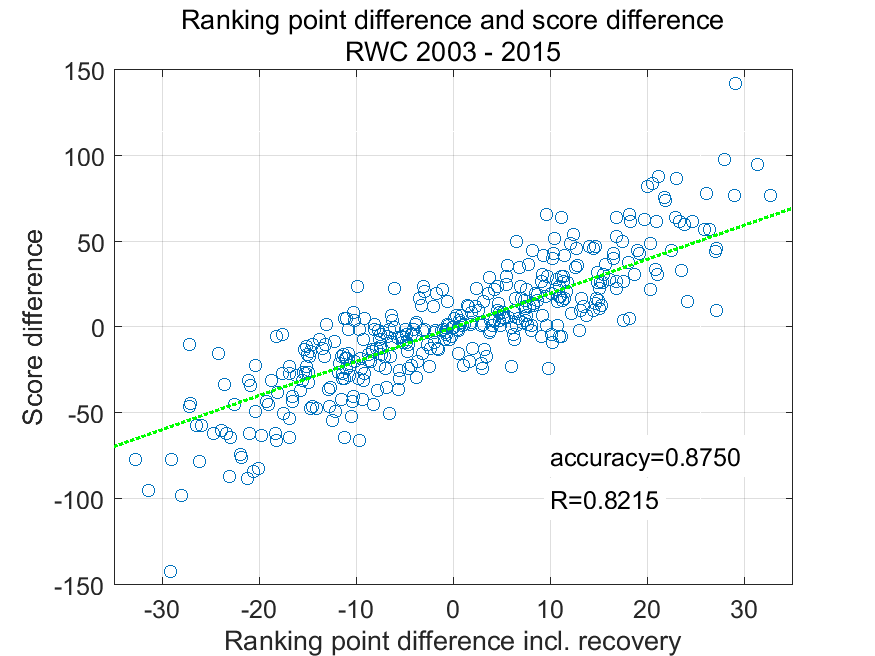In the pool stages in RWC, five possible outcomes, 5, 4, 2, 1, or 0 points, could be awarded after one match. The awarded points are collected from the past 4 RWC tournamtns.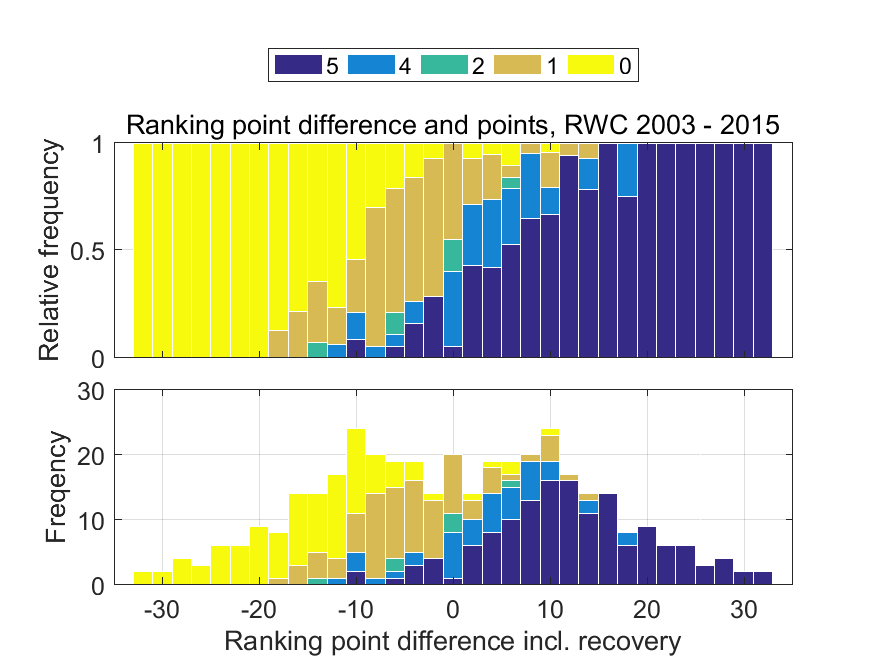The horizontal axis shows the ranking point difference. The vertical axes of the upper and lower panels are relative and absolute frequencies, respectively.

The relative frequencies in the upper panel are fitted by logit model (logistic regression function) whose argument is the ranking point difference. The fitted result is the figure below.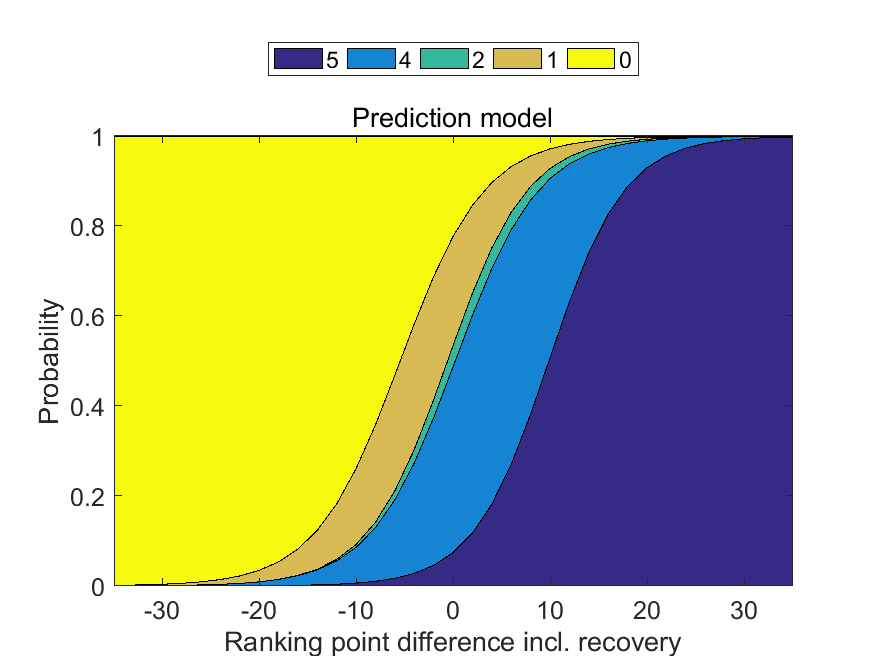The fitted curves are used as the prediction models. The predictions in this article are calculated using these models.